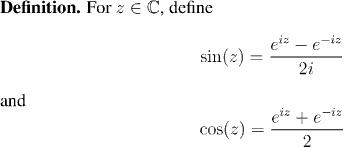Analysis WebNotes
arrow_back arrow_forward

# Class Contents

## Trigonometric Functions

We take our definitions of the trigonometric functions sin(x) and cos(x) from the complex exponential functions.This is quite a far cry from the ratios of sides of triangles, which is how we first learned about these functions. It is natural to ask, "What possible connection do these definitions have with the familar functions of trigonometry?"

The reason we make such a strange-looking definition for these familiar functions goes right to the heart of the philosophy of Analysis, and much of modern mathematics. As we discussed in the Introduction, the aim of Analysis is to set up all of the results in calculus and function-theory on the foundation of numeric and algebraic arguments. It must be possible, ultimately, to trace all of our reasoning back to the axioms of the real numbers. Geometric arguments are invaluable to gain understanding or intuition, but they are not the tools of proof.

There is no place in this scheme of things for a definition of sin(x) and cos(x) in terms of triangles. In fact, even with the whole theory developed so far, we have no formal definitions of anything corresponding to triangles!

So how can such innately geometric objects as the trigonometric functions be described in this context? Although it sounds like we've been taking a very ethereal, philosophic stance, the answer to that question is staunchly practical.

"If it looks like a duck, and it quacks like a duck, then it must be a duck"

That old saying is exactly the right answer to the worries we might have about our definition of the sine and cosine functions. We are going to work with these definitions for a while, and by the end of this section we will have proved that our definitions of sine and cosine have all the same properties that you expect from the geometric definitions. You will see that there is no algebraic test to distinguish the functions defined above from the functions you get by analyzing triangles. The double angle formulae, the derivatives, the periodicity -- everything is the same.

The functions defined above behave exactly as our geometric intuition says that they should. (We can even prove that no other two functions could satisfy all of the properties that these do.) And we can have the satisfaction that our definition is completely analytical. No appeal to geometry, or anything else that can't be related to the real number system, was necessary. So it makes sense to accept this definition for all our applications.

One feature of the definitions we have for sine and cosine is that many of the less familiar properties of the trigonometric functions will fall out of our definition quite easily. Surprisingly, it will be the most geometrically obvious that we have to work hardest for.

We will start with a series of factoids which collect some of the properties which we'll be basing everything else on, before starting to prove the harder results.